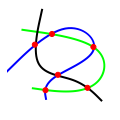CGAL 4.12.1 - 2D Intersection of Curves
2D Intersection of Curves ReferenceBaruch Zukerman, Ron Wein, and Efi Fogel
This package provides three free functions implemented based on the sweep-line paradigm: given a collection of input curves, compute all intersection points, compute the set of subcurves that are pairwise interior-disjoint induced by them, and check whether there is at least one pair of curves among them that intersect in their interior.

Introduced in: CGAL 2.4
Depends on: 2D Arrangements
BibTeX: cgal:wfz-ic2-18b

This chapter describes three functions implemented using the sweep-line algorithm: given a collection $${\mathcal C}$$ of planar curves, compute all intersection points among them, obtain the set of maximal pairwise interior-disjoint subcurves of the curves in $${\mathcal C}$$, or check whether there is at least one pair of curves in $${\mathcal C}$$ that intersect in their interior.

The first two operations are performed in an output-sensitive manner.

## Functions

• CGAL::compute_intersection_points
• CGAL::compute_subcurves
• CGAL::do_curves_intersect

## Functions

template<class InputIterator , class OutputIterator >
OutputIterator CGAL::compute_intersection_points (InputIterator curves_begin, InputIterator curves_end, OutputIterator points, bool report_endpoints=false)
Given a range of curves, compute all intersection points between two (or more) input curves. More...

template<class InputIterator , class OutputIterator , class Traits >
OutputIterator CGAL::compute_intersection_points (InputIterator curves_begin, InputIterator curves_end, OutputIterator points, bool report_endpoints=false, Traits traits)
Given a range of curves, compute all intersection points between two (or more) input curves. More...

template<class InputIterator , class OutputIterator >
OutputIterator CGAL::compute_subcurves (InputIterator curves_begin, InputIterator curves_end, OutputIterator subcurves, bool multiple_overlaps=false)
Given a range of curves, compute all $$x$$-monotone subcurves that are pairwise disjoint in their interior, as induced by the input curves. More...

template<class InputIterator , class OutputIterator , class Traits >
OutputIterator CGAL::compute_subcurves (InputIterator curves_begin, InputIterator curves_end, OutputIterator subcurves, bool multiple_overlaps=false, Traits traits=Default_traits())
Given a range of curves, compute all $$x$$-monotone subcurves that are pairwise disjoint in their interior, as induced by the input curves. More...

template<class InputIterator >
bool CGAL::do_curves_intersect (InputIterator curves_begin, InputIterator curves_end)
Given a range of curves, check whether there is at least one pair of curves that intersect in their interior. More...

template<class InputIterator , class Traits >
bool CGAL::do_curves_intersect (InputIterator curves_begin, InputIterator curves_end, Traits traits=Default_traits())
Given a range of curves, check whether there is at least one pair of curves that intersect in their interior. More...

## ◆ compute_intersection_points() [1/2]

template<class InputIterator , class OutputIterator >
 OutputIterator CGAL::compute_intersection_points ( InputIterator curves_begin, InputIterator curves_end, OutputIterator points, bool report_endpoints = false )

#include <CGAL/Surface_sweep_2_algorithms.h>

Given a range of curves, compute all intersection points between two (or more) input curves.

When the flag report_endpoints is true, this function reports all the curve endpoints as well. If a curve endpoint is also an intersection point, it is reported once (regardless of the value of the report_endpoints flag). The value-type of InputIterator is a curve type and the value-type of OutputIterator is a point type. The output points are reported in an increasing $$xy$$-lexicographical order.

Examples:
Surface_sweep_2/plane_sweep.cpp.

## ◆ compute_intersection_points() [2/2]

template<class InputIterator , class OutputIterator , class Traits >
 OutputIterator CGAL::compute_intersection_points ( InputIterator curves_begin, InputIterator curves_end, OutputIterator points, bool report_endpoints = false, Traits traits )

#include <CGAL/Surface_sweep_2_algorithms.h>

Given a range of curves, compute all intersection points between two (or more) input curves.

When the flag report_endpoints is true, this function reports all the curve endpoints as well. If a curve endpoint is also an intersection point, it is reported once (regardless of the value of the report_endpoints flag). The Traits type must be a model of the ArrangementTraits_2 concept, such that the value-type of InputIterator is Traits::Curve_2, and the value-type of OutputIterator is Traits::Point_2. The output points are reported in an increasing $$xy$$-lexicographical order.

## ◆ compute_subcurves() [1/2]

template<class InputIterator , class OutputIterator >
 OutputIterator CGAL::compute_subcurves ( InputIterator curves_begin, InputIterator curves_end, OutputIterator subcurves, bool multiple_overlaps = false )

#include <CGAL/Surface_sweep_2_algorithms.h>

Given a range of curves, compute all $$x$$-monotone subcurves that are pairwise disjoint in their interior, as induced by the input curves.

If the flag multiple_overlaps is true, then a subcurve that represents an overlap of $$k$$ input curves is reported $$k$$ times; otherwise, each subcurve is reported only once. The value-type of InputIterator is a curve type, and the value-type of OutputIterator is an $$x$$-monotone curve type.

Examples:
Surface_sweep_2/plane_sweep.cpp.

## ◆ compute_subcurves() [2/2]

template<class InputIterator , class OutputIterator , class Traits >
 OutputIterator CGAL::compute_subcurves ( InputIterator curves_begin, InputIterator curves_end, OutputIterator subcurves, bool multiple_overlaps = false, Traits traits = Default_traits() )

#include <CGAL/Surface_sweep_2_algorithms.h>

Given a range of curves, compute all $$x$$-monotone subcurves that are pairwise disjoint in their interior, as induced by the input curves.

If the flag multiple_overlaps is true, then a subcurve that represents an overlap of $$k$$ input curves is reported $$k$$ times; otherwise, each subcurve is reported only once. The Traits type must be a model of the ArrangementTraits_2 concept, such that the value-type of InputIterator is Traits::Curve_2, and the value-type of OutputIterator is Traits::X_monotone_curve_2.

## ◆ do_curves_intersect() [1/2]

template<class InputIterator >
 bool CGAL::do_curves_intersect ( InputIterator curves_begin, InputIterator curves_end )

#include <CGAL/Surface_sweep_2_algorithms.h>

Given a range of curves, check whether there is at least one pair of curves that intersect in their interior.

The function returns true if such a pair is found, and false if all curves are pairwise disjoint in their interior. The value-type of InputIterator is a curve type.

Examples:
Surface_sweep_2/plane_sweep.cpp.

## ◆ do_curves_intersect() [2/2]

template<class InputIterator , class Traits >
 bool CGAL::do_curves_intersect ( InputIterator curves_begin, InputIterator curves_end, Traits traits = Default_traits() )

#include <CGAL/Surface_sweep_2_algorithms.h>

Given a range of curves, check whether there is at least one pair of curves that intersect in their interior.

The function returns true if such a pair is found, and false if all curves are pairwise disjoint in their interior. The Traits type must be a model of the ArrangementTraits_2 concept, such that the value-type of InputIterator is Traits::Curve_2.